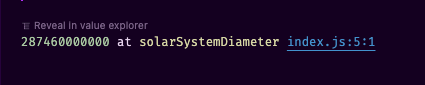# const - JS | lectureNumbers, Dates, Intl & Timers

Numeric Separators

Starting from ES2021, we can use a feature called "Numeric Separators" to format numbers in a way that is easier for us or other developers to read and understand.

Let's say that we wanted to represent a large number—for example, the diameter of our solar system.

script.js
``const solarSystemDiameter = 287460000000;``

Just by looking at this number, I already find it difficult to read and understand. It's not clear what the number represents, and it's not clear what the units are. There are just too many zeros.

Now to help with this, when we write such a large number in standard English, we usually use a thousand separator like the comma. Which then makes it easier for us to read and understand.

``287,460,000,000``

So, how can we do this in JavaScript? Well, we can do this in JavaScript using numeric separators. Numeric seperators are simply underscores that we can place anywhere that we want in our numbers, and which will make it really easy to parse large numbers.

script.js
``const solarSystemDiameter = 287_460_000_000;``

You can now see that by writing this number in this way, it makes it really easy to understand that the above number means, in fact, 287 billion.

Now let's log it to the console to see what JavaScript sees.

script.js
``console.log(solarSystemDiameter);``We can see that the engine ignores the underscores. It simply sees the number itself. And so what this means is that we can place the underscores anywhere that we want.

Now, there are some restrictions as to where we can place the underscores. We can't place them at the beginning or at the end of the number. And we can't place them next to a decimal point. So, for example, the following is not valid.

script.js
``````console.log(3.14_15); // 3.1415
console.log(3_.1415); // SyntaxError
console.log(3._1415); // SyntaxError
console.log(_3.1415); // SyntaxError
console.log(3.1415_); // SyntaxError``````

One final detail we need to be aware of is that when we try to convert a string containing underscores to a number, that will not work as expected.

script.js
``````console.log(Number('22163')); // 22163
console.log(Number('22_163')); // NaN``````

What this means is that you should only use these numeric separators when you are writing down numbers in your code.

If you need to store a number in a string, for example, in an API, or if you get a number as a string from an API, you should not use underscores there, because JavaScript will not be able to parse the number correctly out of that string. It's not going to work as expected, and you will get it's not a number that might introduce bugs into your application.

The same is true with the `parseInt` function.

script.js
``console.log(parseInt('22_163')); // 22``

We do not get `NaN` but 22 instead.